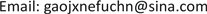1大连海事大学道路与桥梁工程研究所，辽宁 大连

2中铁建大桥工程局集团一公司，辽宁 大连1. 引言

2. 土工试验及土的各项指标

2.1. 土的含水率

ω = m − m s m s × 100 (1)

2.2. 土的界限含水率

A线方程 I P = 0.73 × ( ω L − 20 ) B线方程 ω L = 50%

Liquid limit plastic limit joint test record tabl

I P = 0.73 × ( ω L − 20 ) (2)

I P = 0.73 × ( 30.1 − 20 ) = 7.373 < 9.43

2.3. 颗粒级配分析试验

C u = d 60 d 10 (3)

C c = d 30 2 d 60 d 10 (4)

Particle analysis result

203523200100
10544284889
5832230472
2256147246
1512121638
0.522470422
0.2532048015
0.0741601605

2.4. 最佳含水率试验

m ω = m 1 + 0.01 ω 0 × 0.01 ( ω − ω 0 ) (5)

ω = ( m m d − 1 ) × 100 (6)

ρ d = ρ 1 + 0.01 ω (7)

5个试件分别以含水率为横坐标，干密度为纵坐标，绘制含水率与干密度的关系曲线如图4，取干密度最大点对应的含水率值为最佳含水率，对应纵坐标值为最大干密度，从数据曲线中得出该土样的最佳含水率为20.5%，最大干密度1.680 g/cm3

2.5. 渗透性试验

k t = 2.3 a L A ( t 2 − t 1 ) lg h 1 h 2 (8)

2.6. 直剪试验

1) 对准剪切容器上下盒，插入固定销，在下盒内放透水石和滤纸，将带有试样的环刀刃向上，对准剪盒口，在试样上放滤纸和透水石，将试样小心地推入剪切盒内。

2) 移动传动装置，使上盒前端钢珠刚好与测力计接触，依次加上传压板、加压框架，安装垂直位移量测装置，测记初始读数。

3) 根据工程实际和土的软硬程度施加各级垂直压力，然后向盒内注水。当试样为非饱和试样时，应在加压板周围包以湿棉花。

Data of variable head permeability test result

16:0616:219006656506.86 × 10−49.91 × 10−35.66 × 10−6
16:2116:369006506366.86 × 10−49.46 × 10−35.41 × 10−6
16:3616:519006366226.86 × 10−49.67 × 10−35.53 × 10−6
16:5217:026009008851.03 × 10−37.30 × 10−36.26 × 10−6
17:0217:126008858721.03 × 10−36.43 × 10−35.52 × 10−6
17:1217:226008728601.03 × 10−36.02 × 10−35.17 × 10−6
17:2317:336009909731.03 × 10−37.52 × 10−36.45 × 10−6
17:3317:436009739601.03 × 10−35.84 × 10−35.01 × 10−6
17:4317:536009609461.03 × 10−36.38 × 10−35.47 × 10−6
17:5618:066007277161.03 × 10−36.62 × 10−35.68 × 10−6
18:0618:166007167051.03 × 10−36.72 × 10−35.77 × 10−6
18:1618:266007056941.03 × 10−36.83 × 10−35.86 × 10−6
18:3518:456009989821.03 × 10−37.02 × 10−36.02 × 10−6
18:4518:556009829671.03 × 10−36.69 × 10−35.74 × 10−6
18:5519:056009679541.03 × 10−35.88 × 10−35.04 × 10−6
19:1719:276009349211.03 × 10−36.09 × 10−35.23 × 10−6
19:2719:376009219091.03 × 10−35.70 × 10−34.89 × 10−6
19:3719:476009098961.03 × 10−36.26 × 10−35.37 × 10−6

4) 施加垂直压力，垂直压力的大小应根据工程要求和土的软硬状态确定，宜按25 kpa、50 kpa、100 kpa、200 kpa或100 kpa、200 kpa、300 kpa、400 kpa施加压力。拔出固定销立即开动秒表，以小于0.02 mm/min的剪切速度进行。

5) 当测力计百分表读数不变或后退时，继续剪切至剪切位移为4 mm时停止，记下破坏值。当剪切过程中测力计百分表无峰值时，剪切至剪切位移达6 mm时停止。

6) 剪切结束，吸去盒内积水，退剪切力和垂直压力，移动压力框架，取出试样，测定含水率。

Δ L = 20 n − R (9)

τ = C R (10)

2.7. 土的固结实验

e i = e 0 − ( 1 + e 0 ) Δ h i h 0 (11)

a v = e i − e i + 1 p i + 1 − p i (12)

E s = 1 + e 0 a v (13)

m v = 1 E s = a v 1 + e 0 (14)

C c 或 C s = e i − e i + 1 lg p i + 1 − lg p i (15)

C v = 0.848 ( h ¯ ) 2 t 90 (16)

h ¯ = h 1 + h 2 4 (17)

h ¯ ——最大排水距离，等于某压力下试样初始与终了高度的平均值之半，cm。

t 90 ——固结度达到90%所需时间s。

3. 小结

1) 部分北方沿海路基土属于粗粒土，含水量高，试验粘土天然含水率为20%，最佳含水率为20.5%，液限为30.1%，塑限为20.67%，塑性指数为9.43%，不均匀系数为8.5，曲率系数为1.86，最大干密度1.680 g∙cm−3，黏土的干密度为1.604 g∙cm−3。可为北方沿海路基土进行沉降计算提供数据参考。

Compression index under various pressure
0 kPa25 kPa50 kPa100 kPa200 kPa400 kPa
e i0.730.700.690.670.640.59
C c 或 C s0.02150.03320. 06640.09970.166

Coefficient of consolidation under various pressure
p kPah 1 (cm)h 2 (cm)h ¯ (cm)t 90 (s)C v (10−3 cm2/s)
2521.96880.9926013.85
501.96881.95620.9816013.60
1001.95621.9330.9726013.30
2001.9331.89530.9576013.02
4001.89531.84810.9366012.49

2) 部分北方沿海路基土抗剪强度低，透水性好，压缩性差，固结速度较快。可为沿海路基土的改良、加固等提供参考。